# Unit Circle Calculator

Created by Hanna Pamuła, PhD candidate
Reviewed by Dominik Czernia, PhD candidate and Jack Bowater
Last updated: Apr 06, 2022

Welcome to the unit circle calculator ⭕. Our tool will help you determine the coordinates of any point on the unit circle. Just enter the angle ∡, and we'll show you sine and cosine of your angle.

If you're not sure what a unit circle is, scroll down and you'll find the answer. The unit circle chart and an explanation on how to find unit circle tangent, sine, and cosine are also here, so don't wait any longer - read on!

## What is a unit circle?

A unit circle is a circle with a radius of 1 (unit radius). In most cases, it is centered at the point (0,0), the origin of the coordinate system.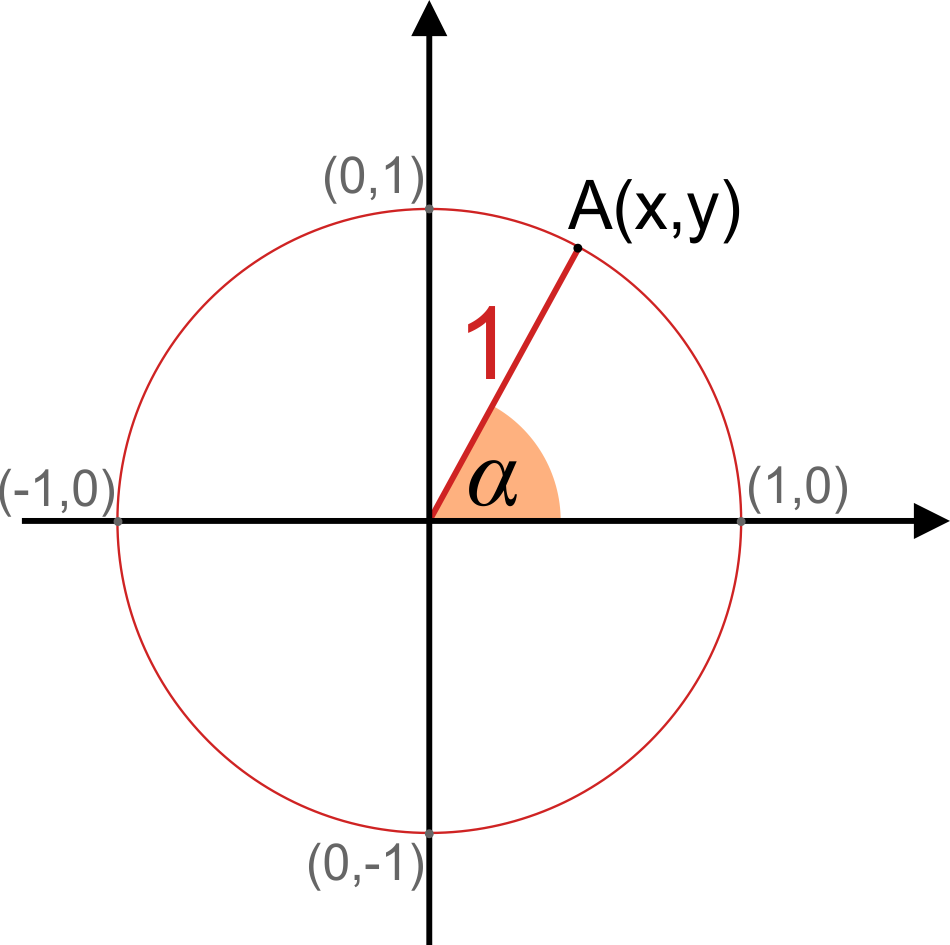Unit circle is a really helpful concept when learning about trigonometry and angle conversion.

Now that you know what a unit circle is, let's proceed to the relations in the unit circle.

## Unit circle: sine and cosine

OK, so why is the unit circle so useful in trigonometry?

TL;DR
Unit circle relations for sine and cosine:

• Sine is the y-coordinate; and
• Cosine is the x-coordinate

Standard explanation:

Let's take any point A on the unit circle's circumference.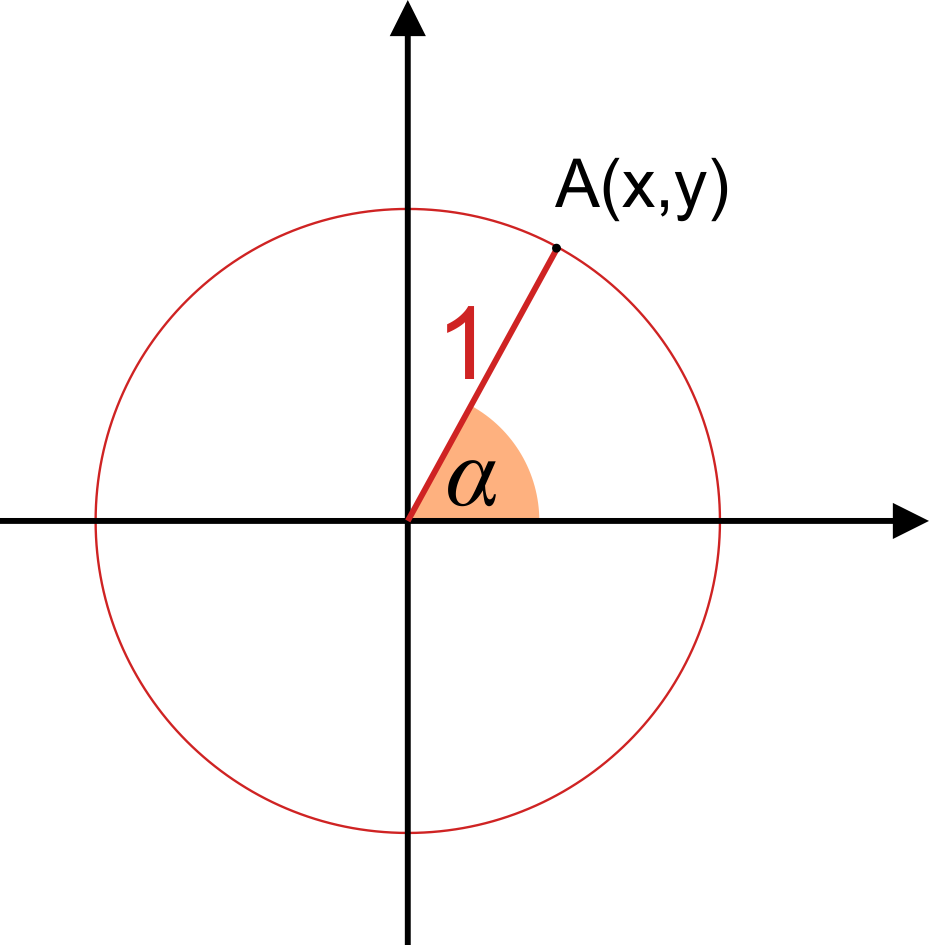• The coordinates of this point are x and y. As it's a unit circle, the radius r is equal to 1 (a distance between point P and center of the circle).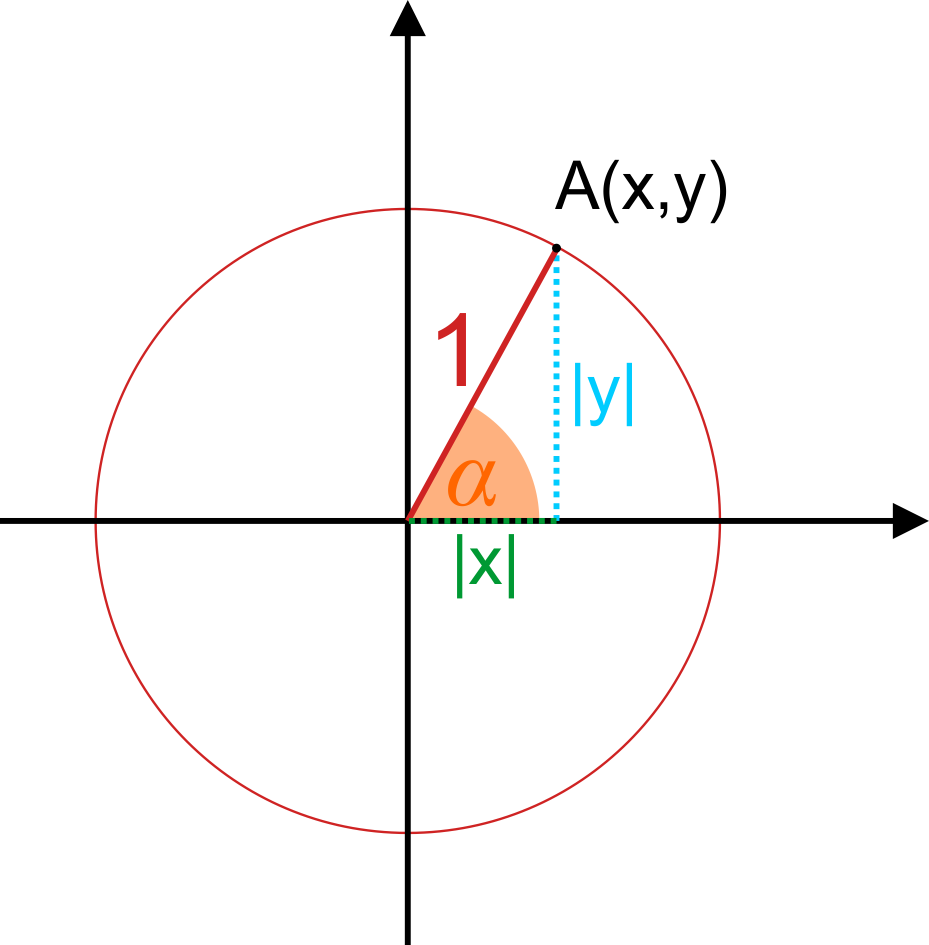• By projecting the radius onto the x and y axes, we'll get a right triangle, where |x| and |y| are the lengths of the legs, and the hypotenuse is equal to 1.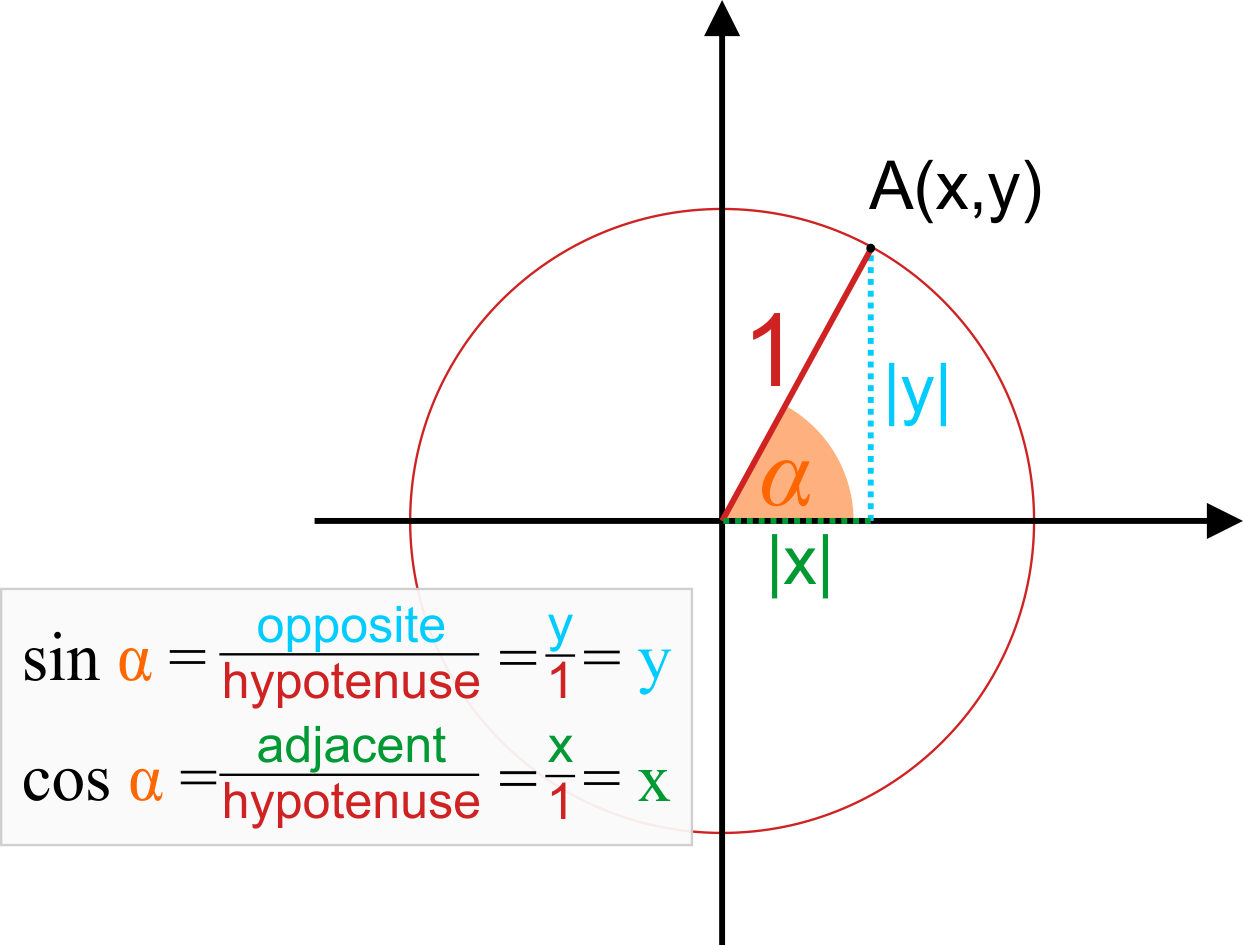• As in every right triangle, you can determine the values of the trigonometric functions by finding the side ratios:
• sin(α) = opposite/hypotenuse = y/1 = y,

So, in other words, sine is the y-coordinate

• cos(α) = adjacent/hypotenuse =

x/1 = x,

and cosine is the x-coordinate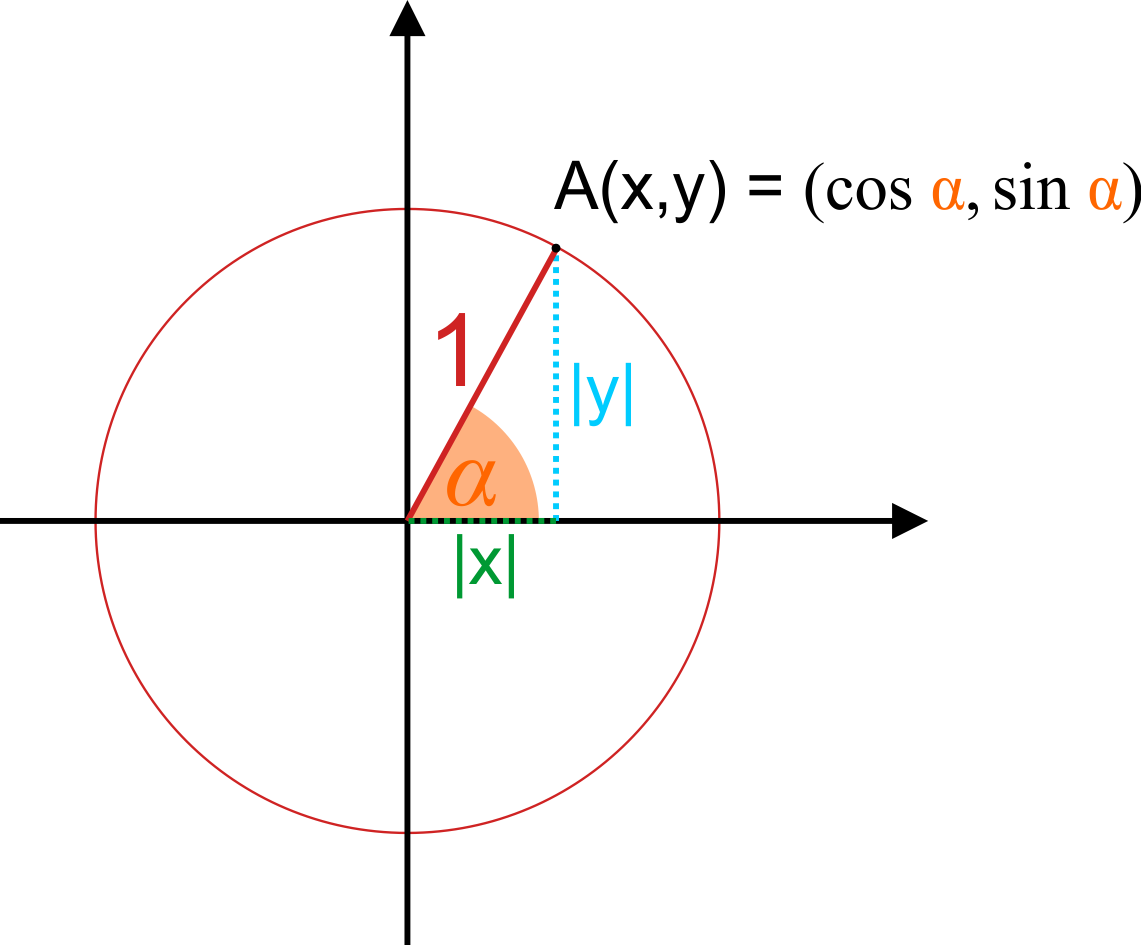The equation of the unit circle, coming directly from the Pythagorean theorem, looks as follows:

x² + y² = 1

or, analogically:

sin²(α) + cos²(α) = 1

## Unit circle tangent & other trig functions

You can find the unit circle tangent value directly if you remember the tangent definition: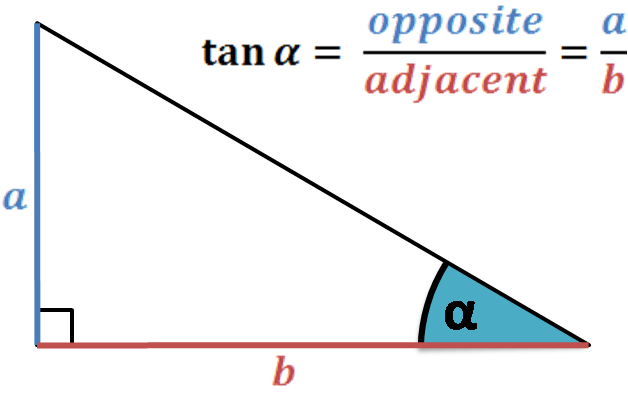The ratio of the opposite and adjacent sides to an angle in a right-angled triangle.

tan(α) = opposite/adjacent

As we learned from , sin(α) = y and cos(α) = x, so:

tan(α) = y/x

We can also define the tangent of the angle as its sine divided by its cosine:

tan(α) = sin(α)/cos(α) = y/x, which of course will give us the same result.

Another method is using our unit circle calculator, of course. 😁

But what if you're not satisfied with just this value, and you'd like to actually to see that tangent value on your unit circle?

It is a bit more tricky than determining sine and cosine - which are simply the coordinates. There are two ways to show unit circle tangent:

Method 1: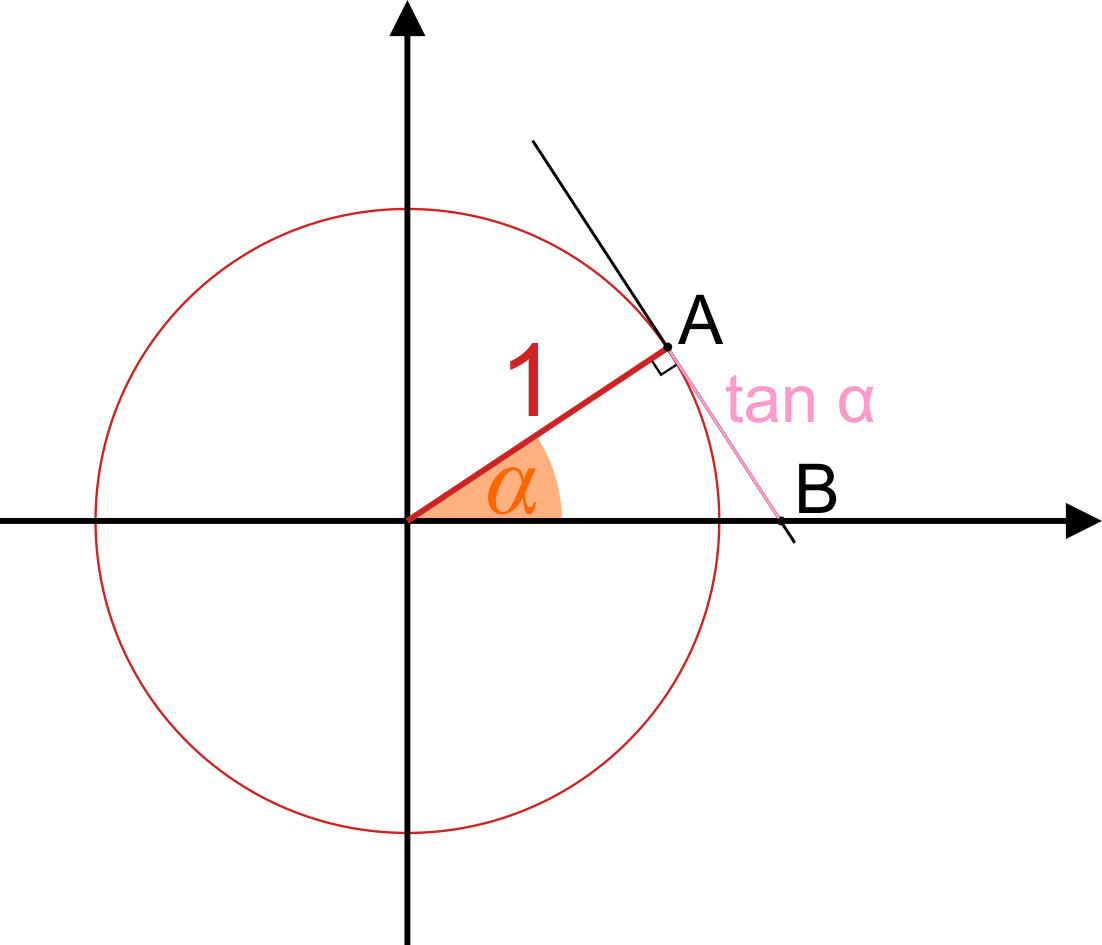1. Create a tangent line at point A.
2. It will intersect the x-axis in point B.
3. The length of the AB segment is the tangent value

Method 2: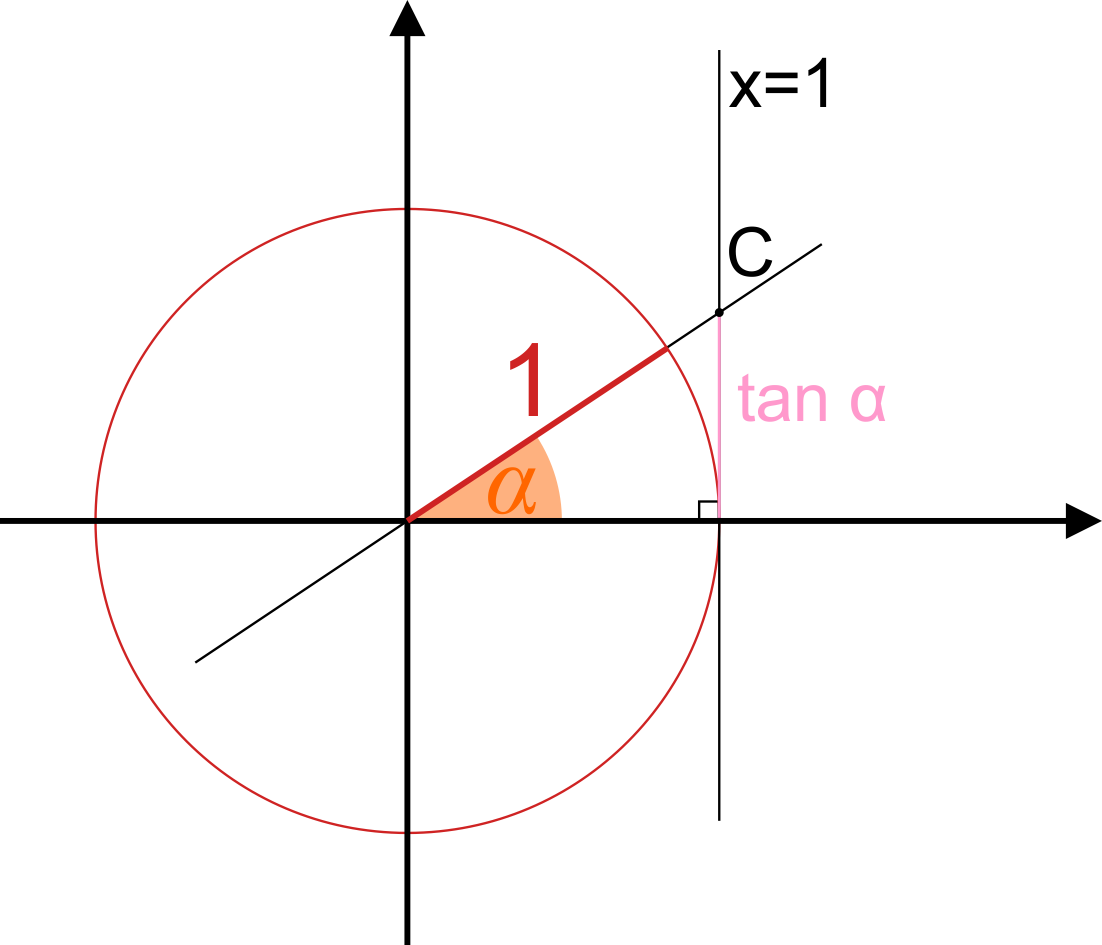1. Draw a line x = 1.
2. Extend the line containing the radius.
3. Name the intersection of these two lines as point C.
4. The tangent, tan(α), is the y-coordinate of the point C.

In both methods we've created right triangles with their adjacent side equal to 1 😎

Sine, cosine and tangent are not the only functions you can construct on the unit circle. Apart from the tangent cofunction - cotangent - you can also present other less known functions, e.g., secant, cosecant, and archaic versine:## Unit circle chart - unit circle in radians and degrees

The unit circle concept is very important because you can use it to the find sine and cosine of any angle. We present some commonly encountered angles in the unit circle chart below:As an example - how to determine sin(150°)?

1. Search for the angle 150°.
2. As we learned before - sine is a y-coordinate, so we take the second coordinate from the corresponding point on the unit circle:

sin(150°) = 1/2

Alternatively, enter the angle 150° to our unit circle calculator. We'll show you the sin(150°) value your y-coordinate, as well as the cosine, tangent, and unit circle chart.

## How to memorize unit circle?

Well, it depends what you want to memorize 🙃 There are two things to remember when it comes to the unit circle:

1. Angle conversion, so how to change between an angle in degrees and one in terms of π (unit circle radians); and

2. The trigonometric functions of the popular angles.

Let's start with the easier first part. The most important angles are those that you'll use all the time:

• 30° = π/6
• 45° = π/4
• 60° = π/3
• 90° = π/2
• 180° = π
• and full angle, 360° = 2π

As these angles are very common, try to learn them by heart ❤️. For any other angle, you can use the formula for angle conversion:

radians = π/180° * degrees

Conversion of the unit circle's radians to degrees shouldn't be a problem anymore! 💪

The other part - remembering the whole unit circle chart, with sine and cosine values - is a slightly longer process. We won't describe it here, but feel free to check out or this . If you prefer watching videos 🖥️ to reading 📘, watch one of these two videos explaining how to memorize the unit circle:

• ; and
• .

Also, this table with commonly used angles might come in handy:

α (angle) Trig functions
deg π rad sin(α) cos(α) tan(α)
30° π/6 1/2 √3/2 √3/3
45° π/4 √2/2 √2/2 1
60° π/3 √3/2 1/2 √3

And if any methods fail, feel free to use our unit circle calculator - it's here for you, forever ❤️ Hopefully, playing with the tool will help you understand and memorize the unit circle values!

Hanna Pamuła, PhD candidateAngle
deg
People also viewed…

### Books vs e-Books